# Using IF function in Excel: formulas for numbers, text, dates, blank cells

IF is one of the most popular and useful functions in Excel. You use an IF statement to test a condition and to return one value if the condition is met, and another value if the condition is not met.

In this tutorial, we are going to learn the syntax and common usages of Excel IF function, and then will have a closer look at formula examples that will hopefully prove helpful both to beginners and experienced users.

## Excel IF function - syntax and usage

The IF function is one of logical functions that evaluates a certain condition and returns the value you specify if the condition is TRUE, and another value if the condition is FALSE.

The syntax for IF is as follows:

IF(logical_test, [value_if_true], [value_if_false])

As you see, the IF function has 3 arguments, but only the first one is obligatory, the other two are optional.

• logical_test (required) - a value or logical expression that can be either TRUE or FALSE. In this argument, you can specify a text value, date, number, or any comparison operator.

For example, your logical test can be expressed as or B1="sold", B1<12/1/2014, B1=10 or B1>10.

• value_if_true (optional) - the value to return when the logical test evaluates to TRUE, i.e. if the condition is met.

For example, the following formula will return the text "Good" if a value in cell B1 is greater than 10: `=IF(B1>10, "Good")`

• value_if_false (optional) - the value to be returned if the logical test evaluates to FALSE, i.e. if the condition is not met.

For example, if you add "Bad" as the third parameter to the above formula, it will return the text "Good" if a value in cell B1 is greater than 10, otherwise, it will return "Bad": `=IF(B1>10, "Good", "Bad")`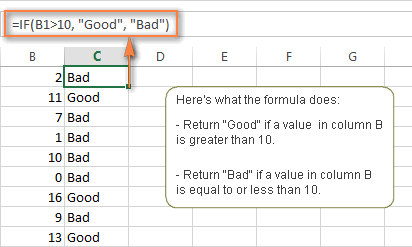#### IF function - things to remember!

Though the last two parameters of the IF function are optional, your formula may produce unexpected results if you don't know the underlying logic beneath the hood.

1. If value_if_true is omitted

If the value_if_true argument is omitted (i.e. there is only a comma following logical_test), the IF function returns zero (0) when the condition is met. Here is an example of such a formula:

`=IF(B1>10,, "Bad")`

In case you don't want your Excel IF statement to display any value when the condition is met, enter double quotes ("") in the second parameter, like this: `=IF(B1>10, "", "Bad")`. Technically, in this case the formula returns an empty string, which is invisible to the user but perceivable to other functions.

The following screenshot demonstrates the above approaches in action, and the second one seems to be more sensible: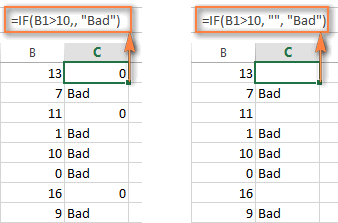2. If value_if_false is omitted

If you don't care what happens when the specified condition is not met, you can omit the 3rd parameter in your formulas, which will result in the following.

If the logical test evaluates to FALSE and the `value_if_false` parameter is omitted (there is just a closing bracket after the `value_if_true` argument), the IF function returns the logical value FALSE. It's a bit unexpected, isn't it? Here is an example of such a formula: `=IF(B1>10, "Good")`

Putting a comma after the value_if_true argument forces your IF statement to return 0, which doesn't make much sense either: `=IF(B1>10, "Good",)`

And again, the most reasonable approach is to put "" in the third argument, in this case you will have empty cells when the condition is not met: `=IF(B1>10, "Good", "")`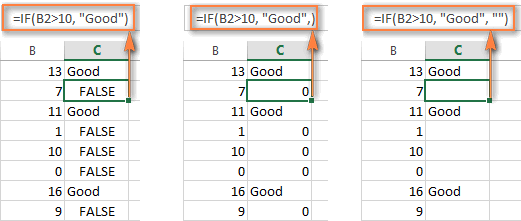3. Get the IF function to display logical values TRUE or FALSE

For your Excel IF formula to display the logical values TRUE and FALSE when the specified condition is met and not met, respectively, type TRUE in the `value_if_true` argument. The `value_if_false` parameter can be FALSE or omitted. Here's a formula example:

`=IF(B1>10, TRUE, FALSE)`
or
`=IF(B1>10, TRUE)`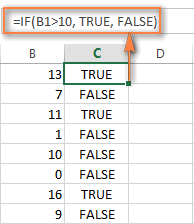Note. For your IF statement to return TRUE and FALSE as the logical values (Boolean values) that other formulas can recognize, make sure you don't enclose them in double quotes. A visual indication of a Boolean is middle align in a cell, as you see in the screenshot above.

If you want "TRUE" and "FALSE" to be usual text values, enclose them in "double quotes". In this case, the returned values will be aligned left and formatted as General. No Excel formula will recognize such "TRUE" and "FALSE" text as logical values.

4. IF statement to perform a math operation and return a result

Instead of returning certain values, you can get your IF formula to test the specified condition, perform a corresponding math operation and return a value based on the result. You do this by using arithmetic operators or other functions in the `value_if_true` and /or `value_if_false` arguments. Here are just a couple of formula examples:

Example 1: `=IF(A1>B1, C3*10, C3*5)`

The formula compares the values in cells A1 and B1, and if A1 is greater than B1, it multiplies the value in cell C3 by 10, by 5 otherwise.

Example 2: `=IF(A1<>B1, SUM(A1:D1), "")`

The formula compares the values in cells A1 and B1, and if A1 is not equal to B1, the formula returns the sum of values in cells A1:D1, an empty string otherwise.

## Using the IF function in Excel - formula examples

Now that you are familiar with the IF function's syntax, let's look at some formula examples and learn how to use it in real-life scenarios.

### Excel IF statement for numbers: greater than, less than, equal to

The use of the IF function with numeric values is based on using different comparison operators to express your conditions. You will find the full list of logical operators illustrated with formula examples in the table below.

 Condition Operator Formula Example Description Greater than > `=IF(A2>5, "OK",)` If the number in cell A2 is greater than 5, the formula returns "OK"; otherwise 0 is returned. Less than < `=IF(A2<5, "OK", "")` If the number in cell A2 is less than 5, the formula returns "OK"; an empty string otherwise. Equal to = `=IF(A2=5, "OK", "Wrong number")` If the number in cell A2 is equal to 5, the formula returns "OK"; otherwise the function displays "Wrong number". Not equal to <> `=IF(A2<>5, "Wrong number", "OK")` If the number in cell A2 is not equal to 5, the formula returns "Wrong number "; otherwise - "OK". Greater than or equal to >= `=IF(A2>=5, "OK", "Poor")` If the number in cell A2 is greater than or equal to 5, the formula returns "OK"; otherwise - "Poor". Less than or equal to <= `=IF(A2<=5, "OK", "")` If the number in cell A2 is less than or equal to 5, the formula returns "OK"; an empty string otherwise.

The screenshot below demonstrates the IF formula with the "Greater than or equal to" logical operator in action: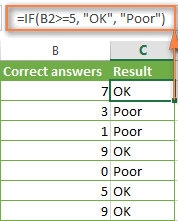### Excel IF examples for text values

Generally, you write an IF statement with text using either "equal to" or "not equal to" operator, as demonstrated in a couple of IF examples that follow.

#### Example 1. Case-insensitive IF formula for text values

Like the overwhelming majority of functions, IF is case-insensitive by default. What it means for you is that logical tests for text values do not recognize case in usual IF formulas.

For example, the following IF formula returns either "Yes" or "No" based on the "Delivery Status" (column C):

`=IF(C2="delivered", "No", "Yes")`

Translated into plain English, the formula tells Excel to return "No" if a cell in column C contains the word "Delivered", otherwise return "Yes". At that, it does not really matter how you type the word "Delivered" in the logical_test argument - "delivered", "Delivered", or "DELIVERED". Nor does it matter whether the word "Delivered" is in lowercase or uppercase in the source table, as illustrated in the screenshot below.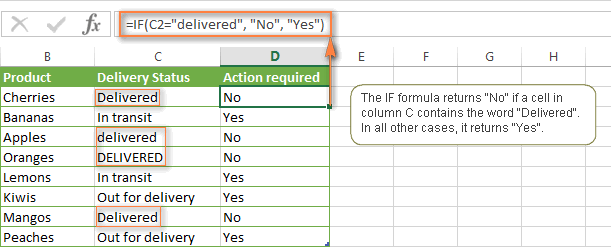Another way to achieve exactly the same result is to use the "not equal to" operator and swap the value_if_true and value_if_false arguments:

`=IF(C2<>"delivered", "Yes", "No")`

#### Example 2. Case-sensitive IF formula for text values

If you want a case-sensitive logical test, use the IF function in combination with EXACT that compares two text strings and returns TRUE if the strings are exactly the same, otherwise it returns FALSE. The EXACT functions is case-sensitive, though it ignores formatting differences.

You use IF with EXACT in this way:

`=IF(EXACT(C2,"DELIVERED"), "No", "Yes")`

Where C is the column to which your logical test applies and "DELIVERED" is the case-sensitive text value that needs to be matched exactly.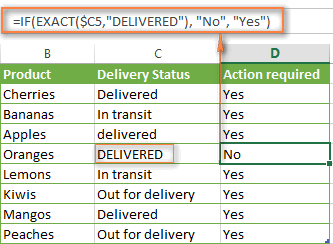Naturally, you can also use a cell reference rather than a text value in the 2nd argument of the EXACT function, if you want to.

Note. When using text values as parameters for your IF formulas, remember to always enclose them in "double quotes".

#### Example 3. Excel IF statement with wildcard (partial match)

If you want to base your condition on a partial match rather than exact match, an immediate solution that comes to mind is using wildcard characters (* or ?) in the logical_test argument. However, this simple and obvious approach won't work. Many functions accept wildcards, but regrettably IF is not one of them.

A solution is to use IF in combination with ISNUMBER and SEARCH (case-insensitive) or FIND (case-sensitive) functions.

For example, if No action is required both for "Delivered" and "Out for delivery" items, the following formula will work a treat:

`=IF(ISNUMBER(SEARCH("deliv",C2)), "No", "Yes")`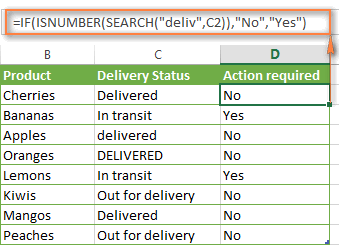We've used the SEARCH function in the above formula since a case-insensitive match suits better for our data. If you want a case-sensitive match, simply replace SEARCH with FIND in this way:

=IF(ISNUMBER(FIND("text", where to search)), value_if_true, value_if_false)

### Excel IF formula examples for dates

At first sight, it may seem that IF formulas for dates are identical to IF statements for numeric and text values that we've just discussed. Regrettably, it is not so.

Unlike many other Excel functions, IF cannot recognize dates and interprets them as mere text strings, which is why you cannot express your logical test simply as >"11/19/2014" or >11/19/2014. Neither of the above arguments is correct, alas.

#### Example 1. IF formulas for dates with DATEVALUE function

To make the IF function recognize a date in your logical test as a date, you have to wrap it in the DATEVALUE function, like this DATEVALUE("11/19/2014"). The complete IF formula may take the following shape:

`=IF(C2<DATEVALUE("11/19/2014"), "Completed", "Coming soon")`

As illustrated in the screenshot below, this IF formula evaluates the dates in column C and returns "Completed" if a game was played before Nov-11. Otherwise, the formula returns "Coming soon".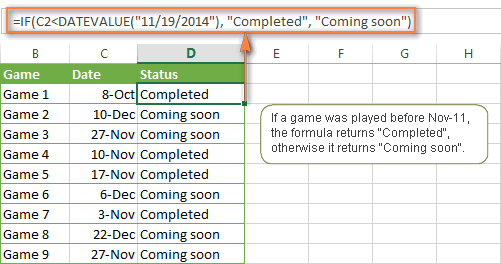#### Example 2. IF formulas with TODAY() function

In case you base your condition on the current date, you can use the TODAY() function in the logical_test argument of your IF formula. For example:

`=IF(C2<DATEVALUE("11/19/2014"), "Completed", "Coming soon")`

Naturally, the Excel IF function can understand more complex logical tests, as demonstrated in the next example.

#### Example 3. Advanced IF formulas for future and past dates

Suppose, you want to mark only the dates that occur in more than 30 days from now. In this case, you can express the logical_test argument as A2-TODAY()>30. The complete IF formula may be as follows:

`=IF(A2-TODAY()>30, "Future date", "")`

To point out past dates that occurred more than 30 days ago, you can use the following IF formula:

`=IF(TODAY()-A2>30, "Past date", "")`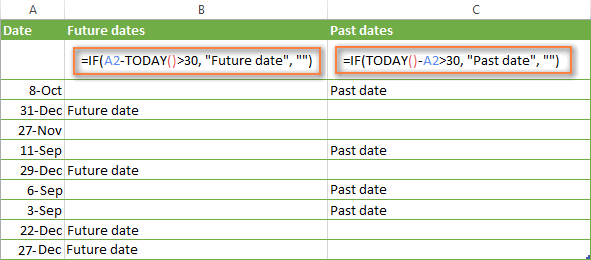If you want to have both indications in one column, you will need to use a nested IF function like this:

`=IF(A2-TODAY()>30, "Future date", IF(TODAY()-A2>30, "Past date", ""))`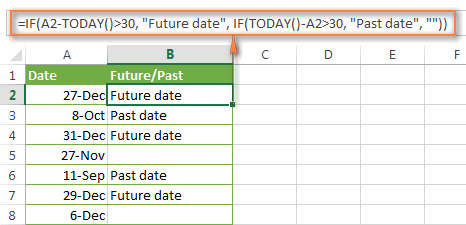### Excel IF examples for blank, non-blank cells

If you want to somehow mark your data based on a certain cell(s) being empty or not empty, you can either:

• Use the Excel IF function in conjunction with ISBLANK, or
• Use the logical expressions ="" (equal to blank) or <>"" (not equal to blank).

The table below explains the difference between these two approaches and provides formula example.

 Logical test Description Formula Example Blank cells ="" Evaluates to TRUE if a specified cell is visually empty, including cells with zero length strings. Otherwise, evaluates to FALSE. `=IF(A1="", 0, 1)` Returns 0 if A1 is visually blank. Otherwise returns 1. If A1 contains an empty string, the formula returns 0. ISBLANK() Evaluates to TRUE is a specified cell contains absolutely nothing - no formula, no empty string returned by some other formula. Otherwise, evaluates to FALSE. `=IF(ISBLANK(A1), 0, 1)` Returns the results identical to the above formula but treats cells with zero length strings as non-blank cells. That is, if A1 contains an empty string, the formula returns 1. Non-blank cells <>"" Evaluates to TRUE if a specified cell contains some data. Otherwise, evaluates to FALSE. Cells with zero length strings are considered blank. `=IF(A1<>"", 1, 0)` Returns 1 if A1 is non-blank; otherwise returns 0. If A1 contains an empty string, the formula returns 0. ISBLANK()=FALSE Evaluates to TRUE if a specified cell is not empty. Otherwise, evaluates to FALSE. Cells with zero length strings are considered non-blank. `=IF(ISBLANK(A1)=FALSE, 0, 1)` Works the same as the above formula, but returns 1 if A1 contains an empty string.

The following example demonstrates blank / non-blank logical test in action.

Suppose, you have a date in column C only if a corresponding game (column B) was played. Then, you can use either of the following IF formulas to mark completed games:

`=IF(\$C2<>"", "Completed", "")`

`=IF(ISBLANK(\$C2)=FALSE, "Completed", "")`

Since there are no zero-length strings in our table, both formulas will return identical results: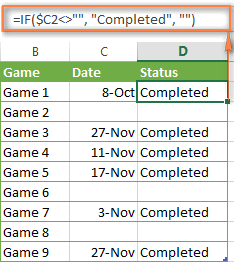Hopefully, the above examples have helped you understand the general logic of the IF function. In practice, however, you would often want a single IF formula to check multiple conditions, and our next article will show you how to tackle this task. In addition, we will also explore nested IF functions, array IF formulas, IFEFFOR and IFNA functions and more. Please stay tuned and thank you for reading!

### 3,842 responses to "Using IF function in Excel: formulas for numbers, text, dates, blank cells"

1.Niels says:

Hi Alexander,
I am trying to get a Region based on two condtions: Animal and Month.
You choose animal in I3 and month in K3.
The list of animals is in D6:D15, the list of months is in E6:E15, the list of regions is in F6:F15.
Multiple animals and months can appear at the same time, in two different regions.
However, the code I am trying to enter does not return the region as I want.
=IF(AND(D6:D15=I3;E6:E15=K3);"ok";"fail")

It's a rather simple code, but I just can't seem to get it to work.

Hope you have an idea. Thanks! :)

•Alexander Trifuntov (Ablebits.com Team) says:

Hello!
I recommend reading in this article how to use the INDEX and MATCH functions to search with multiple criteria.

2.krish says:

hello,
i want to know the formula to calculate the diffrence in time to calculate lateness for staff.
time in is 07:30 am
time out is 17:00 on monday only
time out is 16:45 tuesday to friday
how can i calculate the overtime, lateness and for the early out.
can you please provide me a formula.
Regards,
Krish

•Alexander Trifuntov (Ablebits.com Team) says:

Hello!
Use the information in this manual to calculate reconciliation, late hours, and early exit times.

3.Ebighebi Rowland says:

please I have a challenge using multiple IFS formula when dealing with strings, it returns parse error or #error. the example below:
=IFS(J4>="ON","NOT APPLICABLE",[(J4>="FT","ENUGU DISCO")],[(J4>="LS","ENUGU DISCO")],[(J4>="PG","ENUGU DISCO")],[(J4>="TP","TSP")])

•Alexander Trifuntov (Ablebits.com Team) says:

Remove all extra brackets.

=IFS(J4>="ON","NOT APPLICABLE",J4>="FT","ENUGU DISCO", J4>="LS","ENUGU DISCO",J4>="PG", "ENUGU DISCO",J4>="TP","TSP")

Maybe it will help

4.Ebighebi Rowland says:

THIS SYNTAX BELOW FINALLY WORKED FOR ME:
=IFERROR(IF(J26="ON","ENUGU DISCO",IF(J26="FT","ENUGU DISCO",IF(J26="LS","ENUGU DISCO",IF(J26="PG","ENUGU DISCO",IF(J26="TP","TSP",IF(J26="IT","ENUGU DISCO",IF(J26="ET","TSP"))))))))

ACTUALLY, THE FORMULA IS TO HELP ME DIFFERENTIATE REVENUE LOSES CAUSED BY DIFFERENT FACTORS IN AN ELECTRICITY DISTRIBUTION COMPANY I AM WORKING FOR.
I REALLY APPRECIATE YOUR GUIDANCE OVER HERE.
MORE GRACE TO YOU SIR.

5.Remy says:

Question. Im trying to figure out a formula for my scheduling.
I put the cell as AM/PM/wholeday

AM value is 5
Pm value is 5
Wholeday value is 10
What formula should i use total their hours from monday to sunday. Thank you

•Alexander Trifuntov (Ablebits.com Team) says:

Hello Remy!
I’m sorry but your task is not entirely clear to me. For me to be able to help you better, please describe your task in more detail. Please specify what you were trying to find, what formula you used and what problem or error occurred. Give an example of the source data and the expected result.
It’ll help me understand it better and find a solution for you. Thank you.

6.Jan says:

Hello,
is it somehow possible to have both text and a formula as value_if_true/false? Simple example what I mean and what is wrong =IF(A1-B1=0;"OK";A1-B1 "PIECES MISSING")??
Thanks

•Alexander Trifuntov (Ablebits.com Team) says:

Hello Jan!
You did not describe your problem very accurately. I'll try to guess.
Perhaps you wanted to write down such a formula

=IF(A1-B1=0;"OK";(A1-B1)&" PIECES MISSING")

7.Jon Repp says:

I'm a layman trying to set-up an excel worksheet and hoping someone would be able to tell me how I would write the following formula for a dollar amount;
If cell 7 + cell 9 is > cell 10, then cell 14 = cell 7; If not, then cell 14 = cell 10 - cell 9.

•Alexander Trifuntov (Ablebits.com Team) says:

Hello!
Write this formula in cell A14

=IF((A7+A9)>A10,A7,A10-A9)

Hope this is what you need.

8.EC says:

Hi,
I am trying to return the value of a cell if another cell is greater than an amount - it's a simple one but I cannot figure it out, can you help

•Alexander Trifuntov (Ablebits.com Team) says:

Hello!
You may find this formula useful

=IF(A1>B1,C1,"")

9.Janine says:

Hello!
My knowledge of Excel is basic at best. Is there a way to sort data alphabetically into another worksheet? For example, if I have a list of names on Sheet 1, can I then sort that info into other sheets broken down into parts of the alphabet? EG, Sheet2 = A - H; Sheet3 = I-P; Sheet4 = Q-Z. I'm trying to create a workload list for my staff but their work is divided by alphabet. Thank you.

10.Adao says:

Hello,
I want a formula that check another cell if it contains certain text and just come up with the today's date if find this text.
Eg.: ( if cell A contains ''sent to client'' the result is 27/07/2020)

•Alexander Trifuntov (Ablebits.com Team) says:

Hello!
If I understand your task correctly, the following formula should work for you:

=IF(A3="sent to client",DATE(2020,7,27),"")

or

=IF(A3="sent to client",today(),"")

I hope this will help

11.tusasibwe says:

helo,
if i have multiple figures expected in acell and want to test then, how can i do it?
example in column a, i type 1, 4, 5 9, 17, 23,and 29 an others. i want to be informed in column B that if in A there is 4, it documents y, if 5, its also y and you find that they are around 2000 figures to be tested by y. how can i set it?
many thanks

12.Stuart Howard says:

I have to produce a spreadsheet for covid19 weekly testing of staff. I want to place next due date in cell after entering y in tested cell calculating 7 days ahead from date tested. A1 =date tested, B1 =y for tested C1 = due date by 7 days. Could you assist with formula
Thanks

•James says:

Hi Stuart.

Try the following in cell C1

=IF(B1="Y",A1+7,"")

the "" will show as a blank cell Also dont forget to format cells to dates.

13.James says:

Hi,

I am working on a table for some stats however I am wondering if there is a shorter way using an IF function to return the original value if true.

For Example currently I use.
=IF(SUMIFS(Forming_Bends_Data!\$F\$3:\$F\$50,Forming_Bends_Data!\$A\$3:\$A\$50,Controls!\$A58,Forming_Bends_Data!\$B\$3:\$B\$50,">="&\$C\$24,Forming_Bends_Data!\$B\$3:\$B\$50,"="&\$C\$24,Forming_Bends_Data!\$B\$3:\$B\$50,"<="&\$D\$24))

Im wondering if it is necessary to copy out the original string as these may need to be added to and are getting quite long.

14.Tracey says:

Hello, I am wanting to create a formula that if the value is greater than 0 then the result displays the value but if is is 0 then it displays 'unknown'. Is this possible? Thanks

•Anon Z. says:

IF( A1 > 0, A1, "unknown")

15.Lena Kalyvis says:

hi there, i'm trying to return a value if a date is between two dates, basically trying to sort my data into quarters, so next to my date column i would like a quarter column and want this column formula driven based on a table of dates. is this possible? thank you.

16.Stuart Howard says:

Thanks James. That did what I am looking for. Much appreciated
Stuart

17.Jeff says:

Hi there, I am doing a survey regarding customer satisfaction level to my restaurant. So there are three satisfaction level: A, B, C. I want to find out the problem that leads customer to grade C to our services/foods with precise date. But however I still couldnt make it. Could you please kindly help me out?
The scenario is as below:
Customer Satisfaction Level : A, B, C
Date: 13-08-2020
If "Customer Satisfaction Level:B" , so it should come out with value CSL:B13082020
I tried with IFS formula and it is as below:
=IFS(A2="Customer Satisfaction Level:A", "CSL:A",A2="Customer Satisfaction Level:B","CSL:B",A2="Customer Satisfaction Level:C","CSL:C")
But however, one key problem is how should I key in DATE formula to the value_if_TRUE? Maybe any other formula that can help me on it ?

Your help is very much appreciated.

18.JC says:

I am trying to find the formula for the following:
A. B. C
1. 0. A. D
2. 1. C. A

=IF(A1<1, C1),IF(A1=1, B1)
I am trying to get the cell value if the cell A1 is equal to 1 display cell B1 if value is 0 display cell C1

19.Akshayaa says:

Hi,

I am trying a condition using IF formula, but even the blank cell is counted as value and get the output as "RED" for all the blank cells.
My condition is "GREEN" if the score is 60 or above, "AMBER" if the score is between 50 & 59,"RED" if the score is less then 50.

I tried the below formula; =IF(AND(BO2>=50,BO2<=59),"AMBER",IF(BO2=60,"GREEN",))))

20.SRIKANTH says:

Iuse this function
=IF(C150<DATE(2004,10,1),"250.00","0.00")
so i need to add every date
=date(2004,08,01)
how can i easy to do this.

21.Louise says:

Hi,
I am trying to update a cell with 'Requested', 'Received' or 'Past due' based on 3 other cells with dates in (date requested, due date and date received). I can master 2 statuses but not 3! Please can you help. If there is a date in the 'date received' column this should supersede all other statuses.

22.Lucretia says:

Hi, I want to write a function to change yes/no responses to numbers; So I have yes/no answers to questions and want to do some basic stats (counts etc.) and need to convert the words into numbers (e.g. 0, 1 or 2). Can you please assist?

•Alexander Trifuntov (Ablebits.com Team) says:

Hello!
I’m sorry but your task is not entirely clear to me. For me to be able to help you better, please describe your task in more detail. Do you want to replace text with numbers? This is only possible with a VBA macro. You can set a value in another cell using a formula. What text does the number 0 correspond to? Give an example of the source data and the expected result.
It’ll help me understand it better and find a solution for you. Thank you.

23.Arun A R says:

How to use if function in between the numbers. Eg 8am to 8pm peak, and 8pm to 8am off-peak. What is the formula

•Alexander Trifuntov (Ablebits.com Team) says:

Hello!
If I understand your task correctly, the following formula should work for you:

=IF(AND(C1*24>8,C1*24<20),"peak","off-peak")

Hope this is what you need.

24.Gareth Gittoes says:

I have 2 spreadsheets which are orders and deliveries.
I want to have the order sheet updated with a Y in the received column when the delivery is received.
Can I do this through a IF formula?

•Alexander Trifuntov (Ablebits.com Team) says:

Hello!
Without seeing your data it hard to give you advice. If your data is in 2 different tables, then you most likely need to use the VLOOKUP function.
Give an example of the source data and the expected result.
It’ll help me understand it better and find a solution for you.

25.Chitambuli says:

hie i wanted if its possible to use the IF function in excel to check whether in a particular column the cell have data that is in cell format or not. all the function i have tried so far give a specific date. i just want it to verify if the cell had data that in date format that's it.

•Alexander Trifuntov (Ablebits.com Team) says:

Hello!
To check if a cell is written as a date or just a number or text, you can use

=LEFT(@CELL("format",A1),1)="D"

I hope this will help

•Chitambuli says:

Yes this was a great help, thanx

26.Junaid says:

I want to know the formula to use for time that is greater than 3:00:01

•Alexander Trifuntov (Ablebits.com Team) says:

Hello!
I’m sorry but your task is not entirely clear to me. Could you please describe it in more detail? Please specify what you were trying to find. Write an example of the source data and the result you want to get.

27.Liam says:

Hi,
My problem is i have a column full of times in 24hr time and need to categorize these times into 4 different categories in a separate column (2,3,4,5). i cant figure out the IF function to do this.
for example the first category would be times between 0:00:00AM - 6:00:00Am would be category 2
Thanks,

•Alexander Trifuntov (Ablebits.com Team) says:

Hello!
To convert time to number, use the formula

=A1*24

You can use these numbers in the IF function to create conditions.

28.Bee says:

Hi
I'm trying to do a check in a spreadsheet and the the IF function is giving me the incorrect result:
If(D200=AE200,"YES", "NO")
D200 (this is a formula value of running balance D199 + C200) and AE200 (this is sum of F200:AD200). The value is the same in each cell i.e. 6,603.16 - but gives me a NO result.
I've tried adding in VALUE before the cell reference but this still does not give me the correct result.

•Alexander Trifuntov (Ablebits.com Team) says:

Hello!
Your numbers may differ in some decimal place. I recommend using the rounding function. Or you can perform calculations with the precision with which the numbers appear in your spreadsheet. To do this, use File-Options-Advanced-When calculating ...- Set pricision as displayed.

29.Larry Oxford says:

HELLO!
I am trying to write a formula to fill a cell (say T9) with N/A if the cell J9 includes wording "Standard Type I" or "Standard Type II". The field selections in J9 include but are not limited to
MBCI Standard Type I 20yr
MBCI Standard Type II 20yr
MBCI Single Soucre III 20yr
and so on

•Alexander Trifuntov (Ablebits.com Team) says:

Hello!
Your condition can be written into a formula

=IF(OR(ISNUMBER(FIND("Standard Type I",J9,1)),ISNUMBER(FIND("Standard Type II",J9,1))),"N/A","")

I hope this will help

30.Rajendra Bhatt says:

i have a age list of stuents in column C . age is in yearsmonths and days i.e. 14years,11monts.20 days etc .I wants to calulate under age and over age on a perticular date i.e 01 april 2020. whoes age 1s more then 16 years "overage" and less then 15 year"underage" how can i solve this?

•Alexander Trifuntov (Ablebits.com Team) says:

Hello!
Write an example of the source data and the result you want to get.

31.Rajendra Bhatt says:

i have a age list of stuents in column C . age is in yearsmonths and days i.e. 14years,11monts.20 days etc .I wants to calulate under age and over age on a perticular date i.e 01 april 2020.

32.Epineri Vuli says:

Please assist with this formula, especially with the last criteria. If a student is absent during test week and needs to show up on the Remark Column.
=IF(W5>84,"An excellent performance. Keep it up!.",IF(W5>64,"A very good performance. Can still improve.",IF(W5>49,"A good performance. There is room for improvement.",IF(W5<50,"Needs a lot of improvement.", IF(W5="ab","Was absent during the test week.")))))

•Alexander Trifuntov (Ablebits.com Team) says:

Hello!

=IF(AND(ISNUMBER(W5),W5>84),"An excellent performance. Keep it up!.", IF(AND(ISNUMBER(W5),W5>64),"A very good performance. Can still improve.", IF(AND(ISNUMBER(W5),W5>49),"A good performance. There is room for improvement.", IF(AND(ISNUMBER(W5),W5<50),"Needs a lot of improvement.", IF(W5="ab","Was absent during the test week.","")))))

33.Henry says:

Please i want to know how to use IF function to determine or return only each month names (e.g January , February etc) from a single column of different dates

•Alexander Trifuntov (Ablebits.com Team) says:

Hello!
Your IF formula will be very large. I recommend using the VLOOKUP function to select the name of the month

=VLOOKUP(MONTH(B1), {1,"January";2,"February";3,"March";4,"April";5, "May";6,"June";7,"July";8,"August";9,"September";10, "October";11,"November";12,"December"}, 2,0)

I hope this will help

34.Anon Z. says:

Hi, your explanation and example don't quiet marry up, so I will give you 2 solutions.
Info:
9AM is 09:00 ( 9/24)
5PM is 17:00 (17/24)
From 5PM to 9AM is +1 day -8 hours or +16 hours (16/24) (2/3)
#1 After 5PM show Tomorrow 9AM
IF( ( A1-INT(A1)) > (17/24), INT(A1) +1 +(9/24), A1)
#2 After 17:xx show Tomorrow 09:xx
IF( ( A1-INT(A1)) > (17/24), A1 +(2/3), A1)
# This can get really complex if you want ( 09:00-17:00 M-F, NO Change, Otherwise set time to next work day)

35.Jo says:

I need to work out:

If a number of years worked is less than 5, then 0
If a number of years worked is more than 5, then add 1 for every year above 5

•Alexander Trifuntov (Ablebits.com Team) says:

Hello!
I hope you have studied the recommendations in the above tutorial.
If I understand your task correctly, the following formula should work for you:

=IF(B1<5,0,(B1-5))

36.SHAWNA says:

Hello,
I'd like my IF statement to say:
If AN23=KS, then type in 6/30/2021, otherwise type in 9/28/2020

AN23 is linked to another cell and either says KS or MO
So, the formula should place either 6/30/2021 or 9/28/2020 which are the expiration dates of a state license.

=IF(AN23="KS",DATEVALUE["6/30/2021"], DATEVALUE [9/28/2020])

This is what I typed and it doesn't work.

Thank-you.

•Alexander Trifuntov (Ablebits.com Team) says:

Hello!

=IF(AN23="KS",DATEVALUE("6/30/2021"), DATEVALUE("9/28/2020"))

37.Sharhonda says:

Hello,

I’m having an issue. I’m not sure if this should be a nested IF statements. The first 2 formulas represent the subtraction of dates. The dates can equal 0 to represent no days have passed/a solution was made the same day. I do not want the 0 to populate, I want the cell to remain blank.

1. =IF(ISBLANK(N10),"",(N10-F10)) This formula subtracts & shows 0 in a following cell. Example 3 formula is calculated properly & all values are showing thereafter in other columns.

2. =IF(N10-F10=0,"",N10-F10) This formula subtracts & removed the 0 (as desired). Example 3 formula is NOT calculated when the 0 is removed. It then throws off 3 calculations for all other columns & rows to follow.

3. = IFERROR(IF(ISBLANK(R10),””,(H10*R10)+(U10*V10)),""). This is the formula of the calculated column that is affected perfectly when Example 1 preceded it (non desired 0 in cell) but horribly when Example 2 preceded it (desired blank cell, no 0). I believe the multiplication is throwing it off & the removal of the 0 in Example 2 is causing it. V10 is used to calculate a formula in W10 ONLY when U10 & V10 have values. They will usually be blank.

Is there a way to put a formula in that will distinguish when to use the “+(U10*V10) like an either or? I only need the second half of that equation when U & V have values. I hope this makes sense...

•Alexander Trifuntov (Ablebits.com Team) says:

Hello!
I don't quite understand your calculations. It would be easier to understand them if you wrote an example of the source data and the expected result. The condition "when U & V have values" can be written as

U10&V10=""

It can be used in an IF function.

•Sharhonda says:

38.Sharhonda says:

What I’m trying to express is a valid #3 Statement so that when #2 or #1 statement goes before it, it doesn’t give me an error in the last column that follows them all. The W column.

So H&R column cells values must be calculated. At all times, which is H10*R10.

When U&V column cells have values I have to add the all values, which is (H10*R10)+(U10*V10). (If U10 holds no value then V10 will be calculated to 0) which is my issue. I don’t want the 0 in the cell.

Example 1 formula is column V5. When this formula is input, I see the 0. Example 3 formula is then placed into column W & the value is properly displayed.

Example 2 formula is an alternative to Example 1 which also goes into column V5. When this formula is input, the 0 is removed in V5 as desired. However this formula clears out all values for column W, & Y which requires it to properly display & it doesn’t.

Is there a way to nest the formula for Example 3 [H10*R10] always but ONLY when U10 & V10 have values will I use: (H10*R10)+U10*V10, so that I can use Example 2 as it is? I think it’s messing up because V10 can equal 0 & when I use a formula to not have it displayed it throws everything in all WYZ columns off. I hope this was clearer😩

39.Sushanth says:

Hi,

if the value is (0-6) = need to calculate the value (number)*5%

if the value is (7-10) = need to calculate the value as (number)*10%

But the difficulty is if my value is 8 means first 6 want to calculate as 6*5% and remaining 2 will need to calculate as 2*10%.

So I want the result as ((6*5%) + (2*10%))

If any possible way to write a formulae for that. please guide me.

•Alexander Trifuntov (Ablebits.com Team) says:

Hello!
I hope you have studied the recommendations in the above tutorial.

=IF(A1=8,6*0.05+2*0.1,IF(AND(A1>0,A1<=6),A1*0.05,IF(AND(A1>=7,A1<=10),A1*0.1,"")))

40.HAROLD COLINA says:

can you help me!

What's the formula if a specific number or text will only appear in the cell?

sample : only number 1, will appear on the cell. IF I PUT OTHER NUMBER IT WILL BECOME ERROR.

THANK YOU

41.Alex says:

HELP!

I'm trying to create a formula that will recognize a negative figure in an answer and if it is negative then add to it, if the answer is not a negative figure to leave the original answer

=IF((AND(U24>N4,U24<0)),(U24+N4),(U24-N4))

•Alexander Trifuntov (Ablebits.com Team) says:

Hello!
It’ll help me understand it better and find a solution for you.

42.Adnan says:

Hi i am trying to create a formula which will distinguish high, critical orders as well as express air delivery methods, Now i only want TRUE in next column if both the columns have any one of the text in them.

Order Priority Ship Mode Customers who are urgent
High Regular Air
High Express Air
Critical Express Air
High Delivery Truck
Critical Regular Air
Medium Express Air
Low Regular Air

I have been trying the simple if function to show only high or critical. but if order priority is medium or low and ship mode is express air. it shows false. kindly solve my problem.

•Alexander Trifuntov (Ablebits.com Team) says:

Hello!
To check if both columns have text, use the formula

=IF(AND(A1<>"",B1<>""),TRUE,FALSE)

or

=IF(AND(ISBLANK(A1),ISBLANK(B1)),FALSE,TRUE)

43.Sophia says:

For the example on Excel IF formula examples for dates:
- If the date is left blank, what would be the formula?

I really hope someone can help me. Thanks! :)

•Alexander Trifuntov (Ablebits.com Team) says:

Hello!
I’m sorry but your task is not entirely clear to me. Could you please describe it in more detail? Give an example of the source data. What result do you want to get? Thank you!